Recurrent Neural Network

By Prof. Seungchul Lee
http://iai.postech.ac.kr/
Industrial AI Lab at POSTECH

# 1. Recurrent Neural Network (RNN)¶

• RNNs are a family of neural networks for processing sequential data

## 1.1. Feedforward Network and Sequential Data¶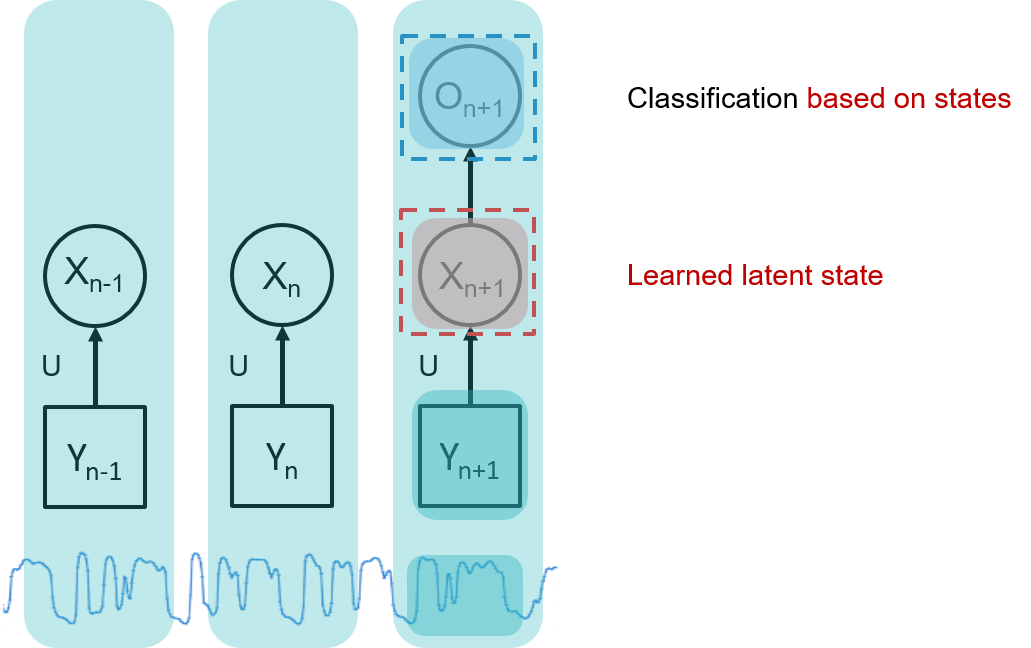• Separate parameters for each value of the time index
• Cannot share statistical strength across different time indices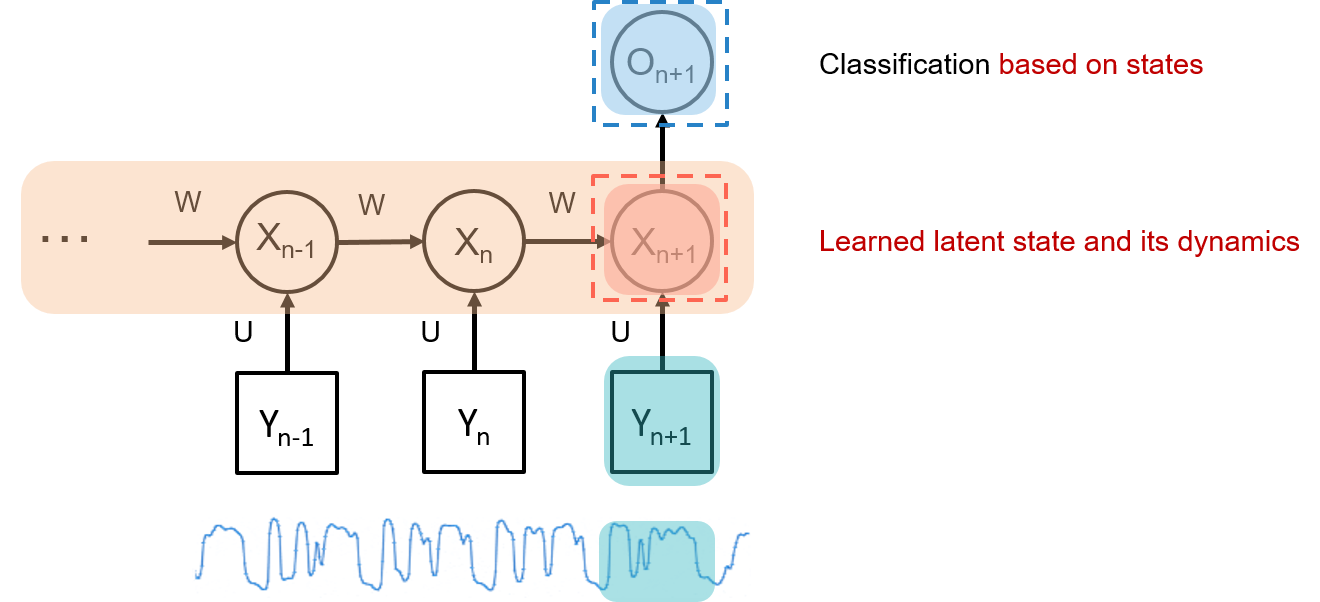In :
%%html
width="560" height="315" frameborder="0" allowfullscreen></iframe></center>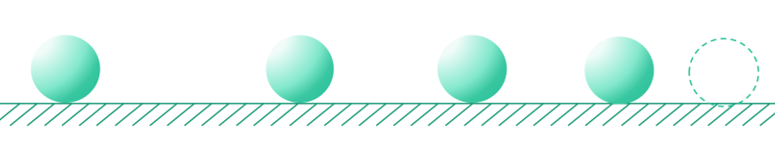## 1.2. Representation Shortcut¶

• Input at each time is a vector
• Each layer has many neurons
• Output layer too may have many neurons
• But will represent everything simple boxes
• Each box actually represents an entire layer with many units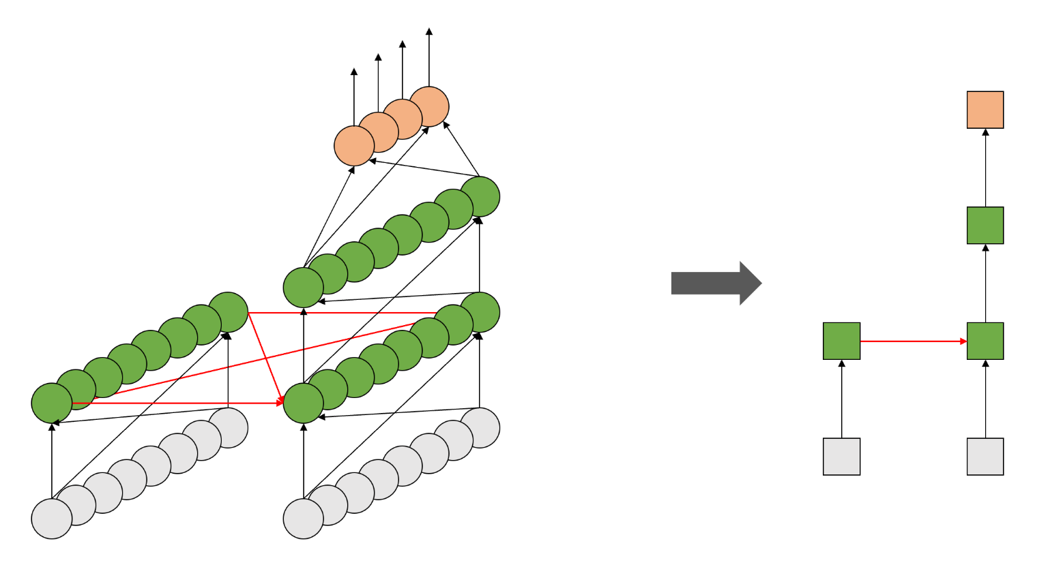## 1.3. Finite-Response Model¶

• Something that happens today only affects the output of the system for $N$ days into the future
• $N$ is the width of the system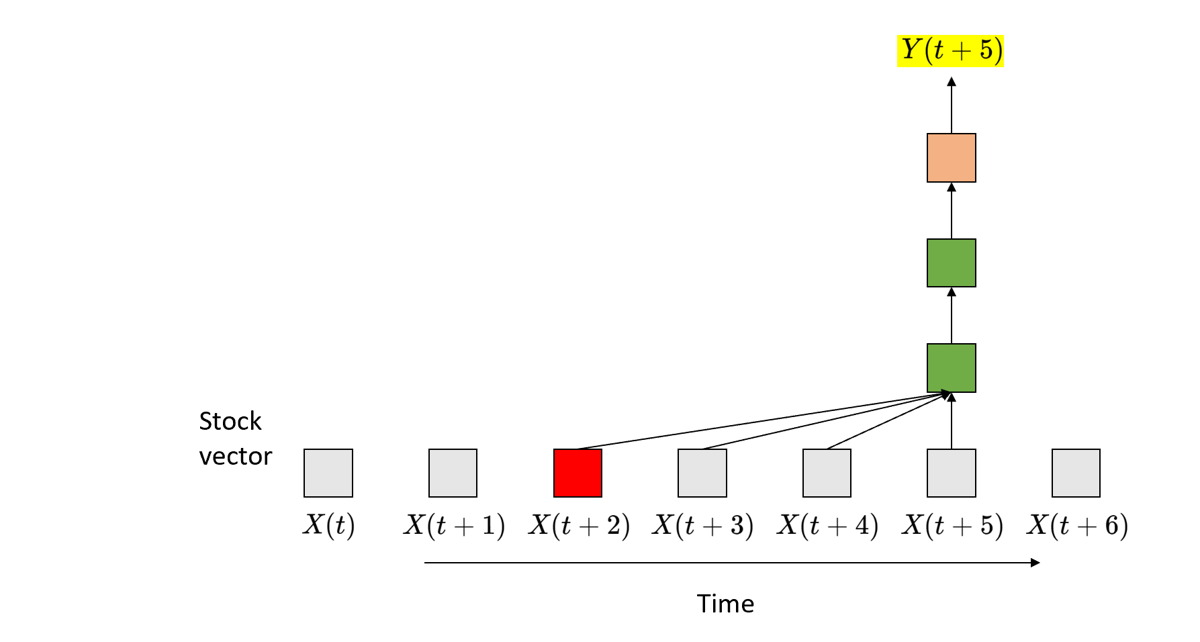• Problem: Increasing the “history” makes the network more complex
$$Y_t = f\left(X_t, X_{t-1}, \cdots, X_{t - N} \right)$$

## 1.4. In Theory, We Want Infinite Memory¶

• Required: Infinite response systems
• What happens today can continue to affect the output forever
• Possibly with weaker and weaker influence
$$Y_t = f\left(X_t, X_{t-1}, \cdots, X_{t - \infty} \right)$$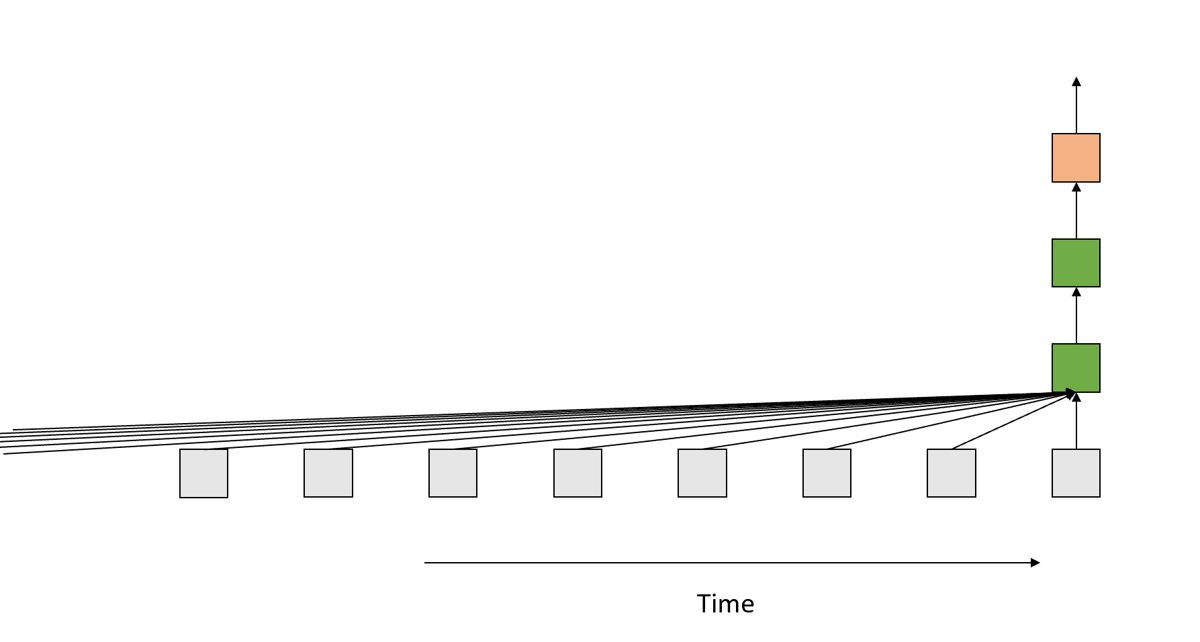• Recursive
• Output contains information about the entire past
$$Y_t = f\left(X_t, X_{t-1}, \cdots, X_{t - \infty} \right) \quad \Longrightarrow \quad Y_t = f\left(X_t, Y_{t-1} \right)$$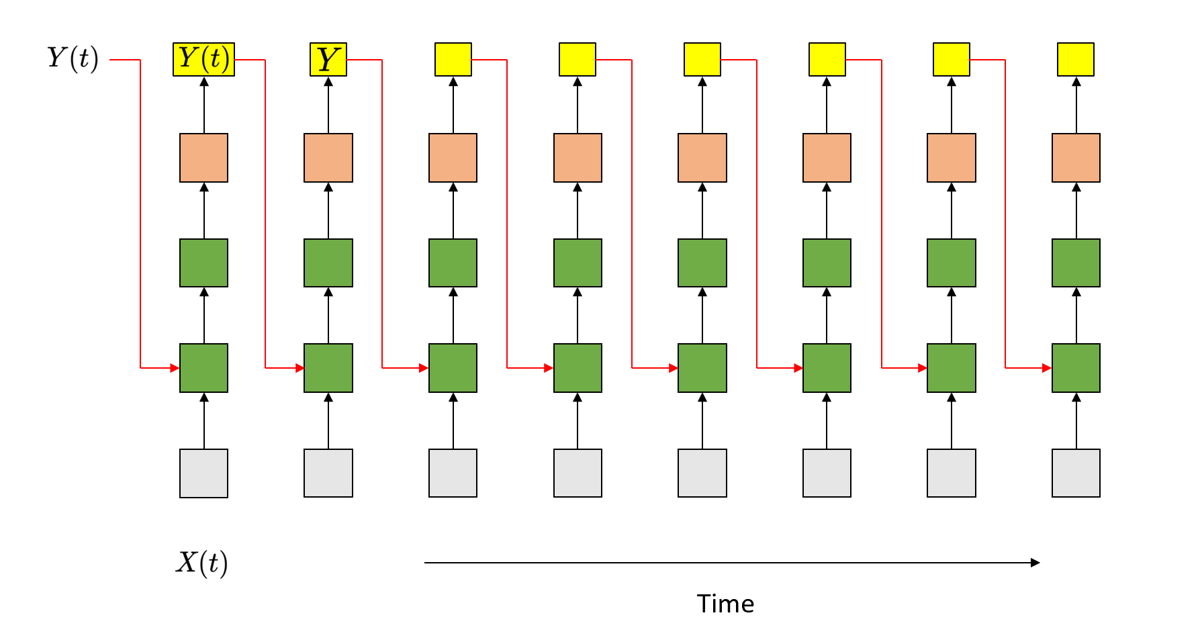## 1.5. An Alternate Model for Infinite Response Systems¶

• The state-space model
\begin{align*} h_t &= f(x_t, h_{t-1})\\ y_t &= g(h_t) \end{align*}
• This is a recurrent neural network
• State summarizes information about the entire past
• Single Hidden Layer RNN (Simplest State-Space Model)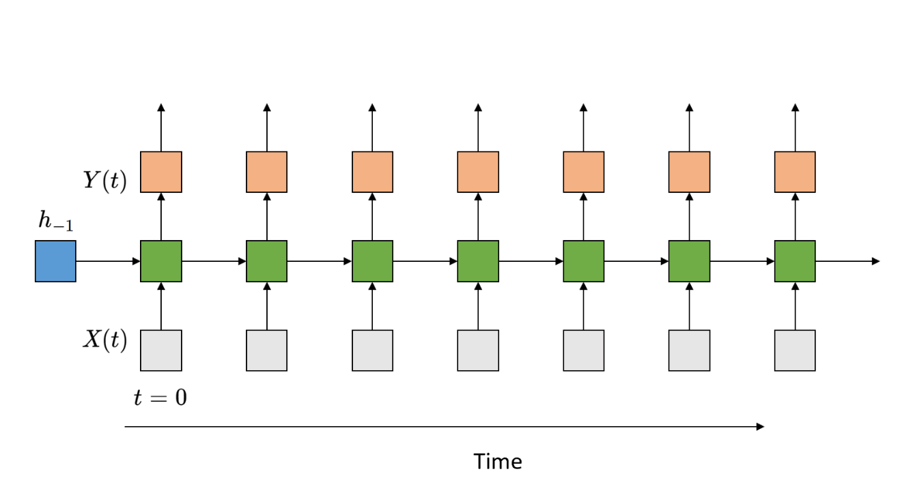• Multiple Recurrent Layer RNN